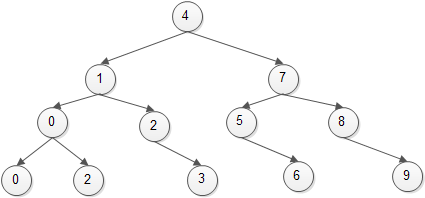# C AVL Tree

Summary: in this tutorial, you will learn about AVL tree and how to implement AVL tree in C.

##Introduction to AVL tree

An AVL tree is a height-balanced binary search tree, where the balance factor is calculated as follows:

Balance Factor = height(left subtree) – height(right subtree)

In an AVL tree, the balance factor of every node is no more than 1. In practice, the balance factor is often stored at each tree’s node. However, a node’s balance factor can be also computed from the heights of its subtrees.

The AVL stands for Adelson-Velskii and Landis, who are the inventors of the AVL tree.

An AVL tree with N nodes, the complexity of any operations including search, insert and delete takes O(logN) time in the average and worst cases. Notice that for the binary search tree, it takes O(N) time in the worst case and O(logN) time in the average case.

In an AVL tree, you may have to re-balance the tree after performing insert and delete operations to keep the tree height-balanced.

## AVL tree implementation in C

AVL tree header file avltree.h:

AVL Tree source code file avltree.c

C AVL tree program main.c

In this tutorial, we have introduced you AVL tree data structure and how to implement AVL tree in C.

• Was this tutorial helpful ?
• YesNo